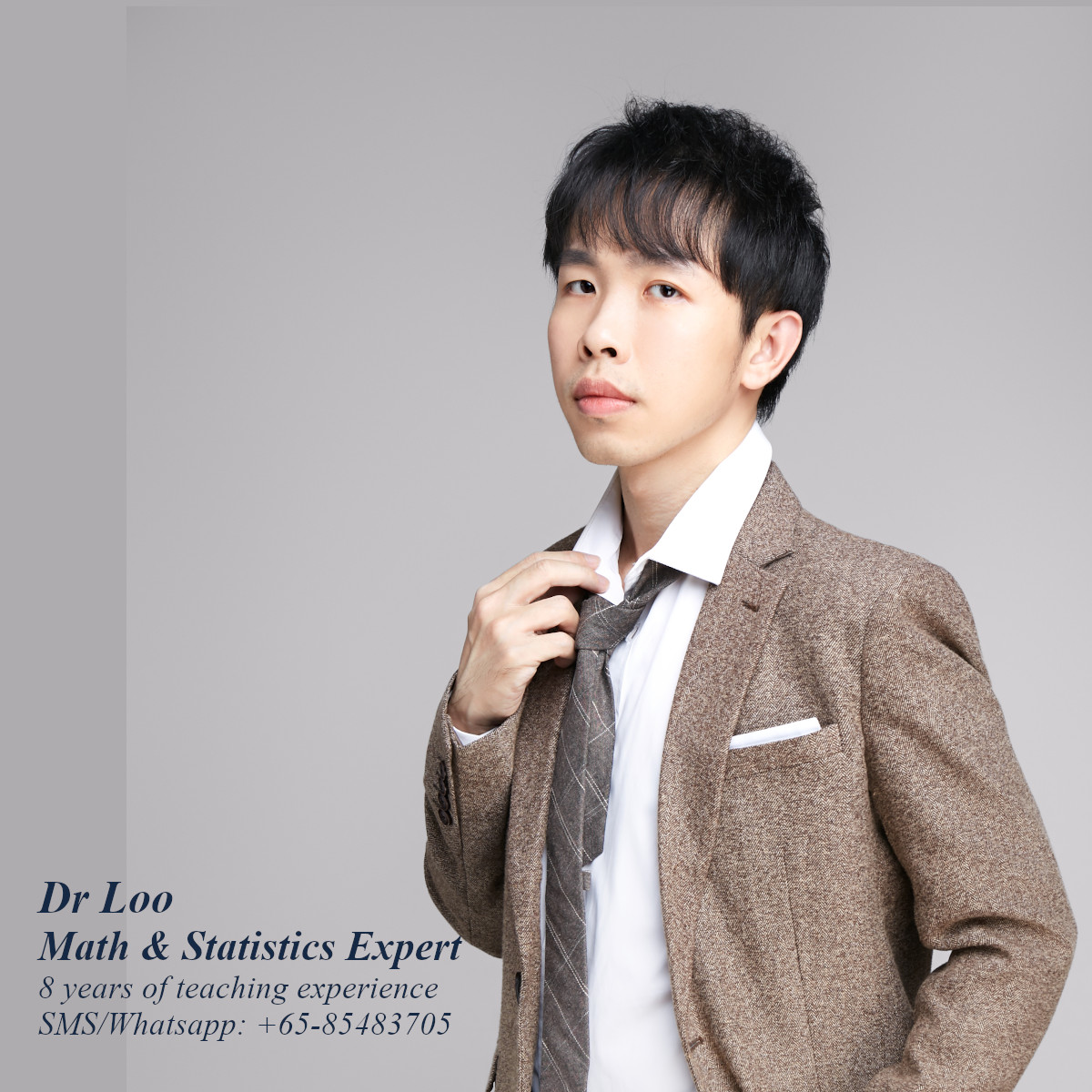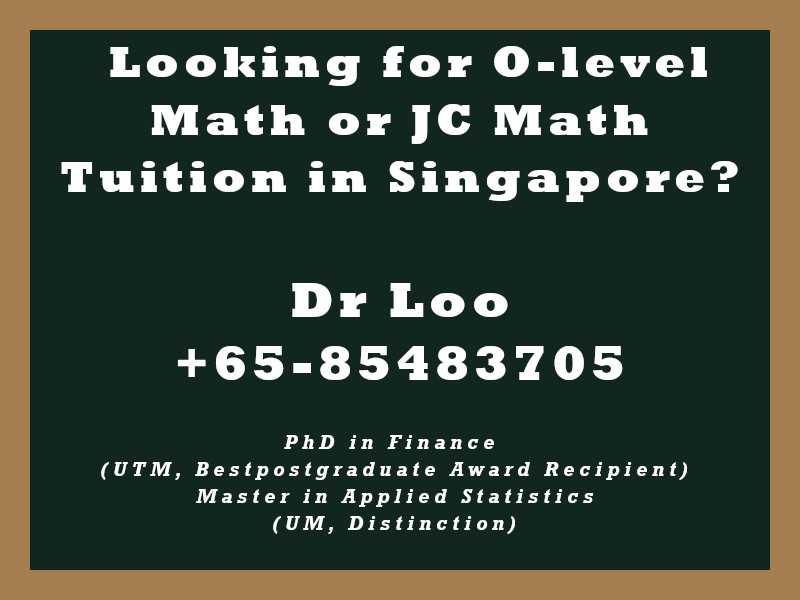+65-85483705

# Centre and Radius of a circle

Question

From the of the circles equations, determine the radius and also the centre.

1. 32 = x2 + y2
2. -30 = x2 + y2 – 6x – 10y

1. Radius = 3, Centre = (0,0)
2. Radius = 2, Centre = (3,5)
Note:

The equation of a circle
(x-h)2 + (y-b)2 = r2
Where
Center = (h,b)

General form of circle equation
ax2 + by2 + cx + dy + e = 0

From General form of circle equation to The equation of a circle:
Perform completing the square on the two variables in the equation.

## About the Singapore Math Tutor - Dr Loo

I am a PhD holder with 9 years of teaching experience on teaching quantitative subjects at secondary school and university levels. My expertise are mathematics, statistics, econometrics, finance and machine learning.

Currently I do provide consultation and tuition for math tuition in Singapore. If you are looking for secondary math tutor in Singapore, JC H2 math tutor or statistics tutor in Singapore, please feel free to contact me at +65-85483705 (SMS/Whatsapp).

Among the Singapore math tuition that I provide, this includes Singapore secondary math tuition (E-math tuition & A-math tuition) and also JC Math Tuition (H1 Math Tuition & H2 Math Tuition). On the other hand, my statistics tuition in Singapore mostly focus on pre-university level until postgraduate level. For those who are looking for online tutoring service, the online O-level math tuition and online A-level math tuition are also available.## For those who are looking for math tution in Singapore

Need help with this topic? I do provide mathematics home tuition in Singapore for O-level math and also JC H2 math. In addition, online math tutoring is available as well. Feel free to contact me if you would like to know further.